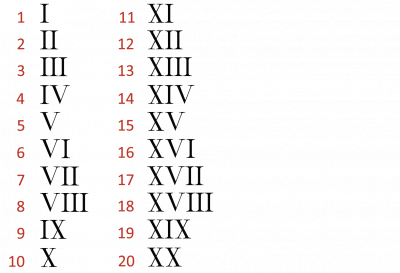The Ancient Romans used Roman numerals as their numbering system.

Roman numerals use capital letters instead of numbers. This might sound complicated but it’s much easier than you think.

• I = 1
• V = 5
• X = 10

If you remember those three numbers you will know how to write up to 20 in Roman numerals!

Take a look at numbers 1 - 20 in Roman numerals.If you put a letter after a larger one it means you add it.

For example:

XVI = 16

This means 10 (X) + 5 (V) + 1 (I) = 16

10 + 5 + 1 = 16

If you put a letter before a larger one it means you take it away.

For example:

IV = 4

This means you take 1 (I) away from 5 (V)

5 − 1 = 4

Now that you have read some examples, let's start the activities!